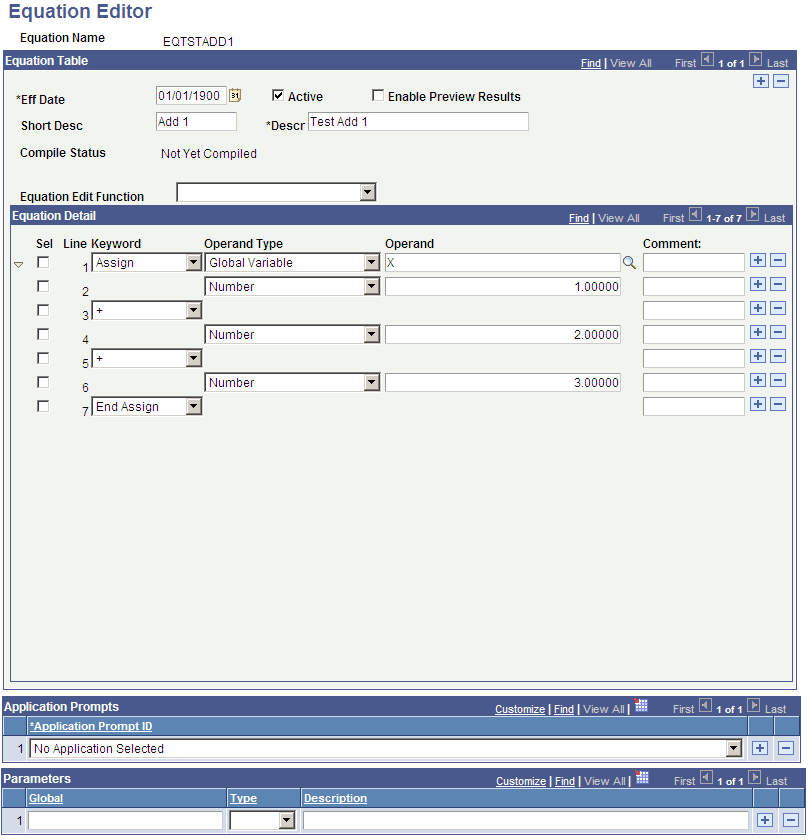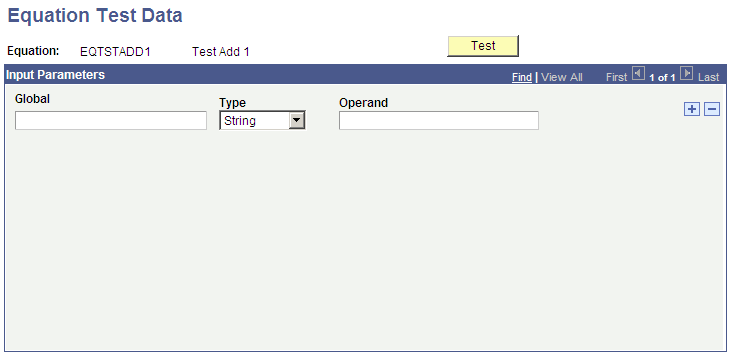Review and Test the Units of Converted Equations

You should test any equation converted to Equation Engine from Equation Processor. This section discusses how to:

• Verify compiled equations.

• Define equation test data.

Note: Financial Aid administrators and Student Financials administrators must complete the steps in this section.

Page Name

Definition Name

Usage

Equation Editor

EQUATION_EDITOR

select Set Up SACR, then select Common Definitions, then select Equation Engine, then select Equation Editor

Verify compiled equations.

Equation Test Data

EQTN_TEST_DATA

select Set Up SACR, then select Common Definitions, then select Equation Engine, then select Equation Test Data

Define equation test data.

Access the Equation Editor page (select Set Up SACR, then select Common Definitions, then select Equation Engine, then select Equation Editor).

Image: Equation Editor page

This example illustrates the fields and controls on the Equation Editor page. You can find definitions for the fields and controls later on this page.Each administrator must review the equation names that he or she entered in the equation conversion setup tables prior to the upgrade.

For each equation, verify that it is compiled. If the equation is not compiled, you must edit it and compile it.

Access the Equation Test Data page (select Set Up SACR, then select Common Definitions, then select Equation Engine, then select Equation Test Data).

Image: Equation Test Data page

This example illustrates the fields and controls on the Equation Test Data page. You can find definitions for the fields and controls later on this page.You can enter test data on this page. After the test data is entered, access the Equation Editor page and click the Test button.

Check the messages that the system provides to verify correct results of the equation test. If test data is missing, the message will identify what is missing.

Financial Aid administrators should carefully review any equations that reference the view FAN_AWD_PER_VW. Specifically, verify whether an AWARD_PERIOD of B (both) is being handled properly. If you want the system to use just the academic award period, you must decide whether you want it used as the first available award period (even if it is the nonstandard award period), or whether you want the system to return a total or maximum of a field. PeopleSoft Campus Solutions provides an alternate view called SFA_PKAWDPER_VW that you can use to return a total or maximum for an award period value of B (both) in addition to existing academic and nonstandard award period rows.